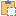#### SpatialElementGeometryCalculator Class

Use this class to calculate the geometry of a spatial element and obtain the relationships between the geometry and the element's boundary elements.

Namespace: Autodesk.Revit.DB
Assembly: RevitAPI (in RevitAPI.dll) Version: 2015.0.0.0 (2015.0.0.0)
Since: 2012

# Syntax

C#
``public class SpatialElementGeometryCalculator : IDisposable``
Visual Basic
``````Public Class SpatialElementGeometryCalculator _
Implements IDisposable``````
Visual C++
``public ref class SpatialElementGeometryCalculator : IDisposable``

# Remarks

This class maintains an internal cache for geometry it has already processed. If you intend to calculate geometry for several elements in the same project you should use a single instance of this class. Note that the cache will be cleared when any change is made to the document.

# ExamplesCopy C#
``````// Calculate a room's geometry and find its boundary faces
SpatialElementGeometryCalculator calculator = new SpatialElementGeometryCalculator(doc);
SpatialElementGeometryResults results = calculator.CalculateSpatialElementGeometry(room); // compute the room geometry
Solid roomSolid = results.GetGeometry(); // get the solid representing the room's geometry
foreach (Face face in roomSolid.Faces)
{
double faceArea = face.Area;
IList<SpatialElementBoundarySubface> subfaceList = results.GetBoundaryFaceInfo(face); // get the sub-faces for the face of the room
foreach (SpatialElementBoundarySubface subface in subfaceList)
{
if (subfaceList.Count > 1) // there are multiple sub-faces that define the face
{
double subfaceArea = subface.GetSubface().Area; // get the area of each sub-face
// sub-faces exist in situations such as when a room-bounding wall has been
// horizontally split and the faces of each split wall combine to create the
// entire face of the room
}
}
}``````Copy VB.NET
``````' Calculate a room's geometry and find its boundary faces
Dim calculator As New SpatialElementGeometryCalculator(doc)
Dim results As SpatialElementGeometryResults = calculator.CalculateSpatialElementGeometry(room)
' compute the room geometry
Dim roomSolid As Solid = results.GetGeometry()
' get the solid representing the room's geometry
For Each face As Face In roomSolid.Faces
Dim faceArea As Double = face.Area
Dim subfaceList As IList(Of SpatialElementBoundarySubface) = results.GetBoundaryFaceInfo(face)
' get the sub-faces for the face of the room
For Each subface As SpatialElementBoundarySubface In subfaceList
If subfaceList.Count > 1 Then
' there are multiple sub-faces that define the face
' get the area of each sub-face
' sub-faces exist in situations such as when a room-bounding wall has been
' horizontally split and the faces of each split wall combine to create the
' entire face of the room
Dim subfaceArea As Double = subface.GetSubface().Area
End If
Next
Next``````

# Inheritance Hierarchy

System Object
Autodesk.Revit.DB SpatialElementGeometryCalculator